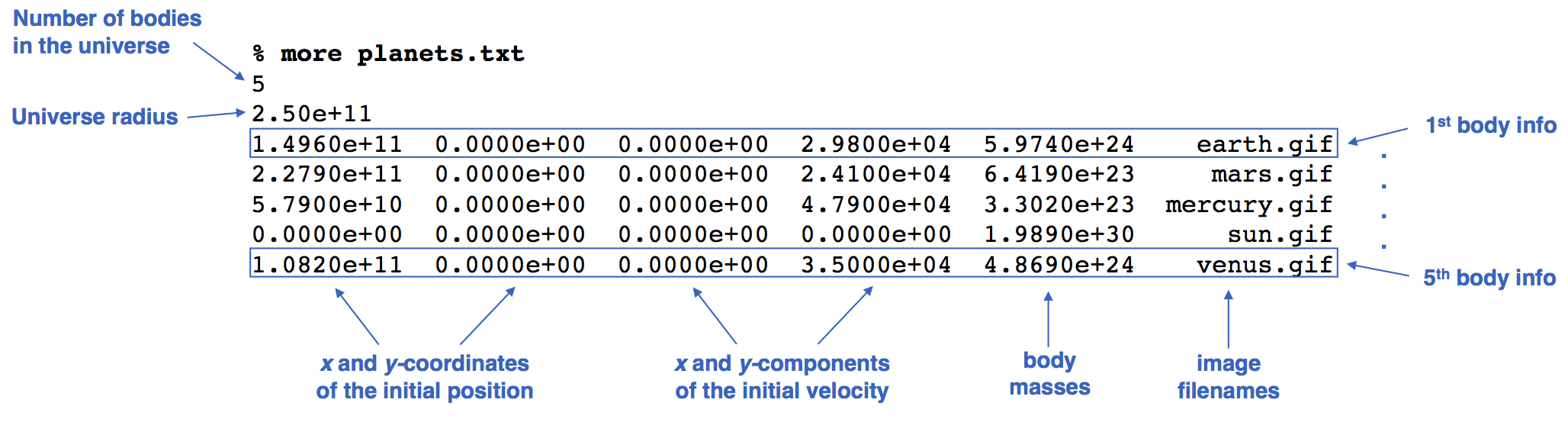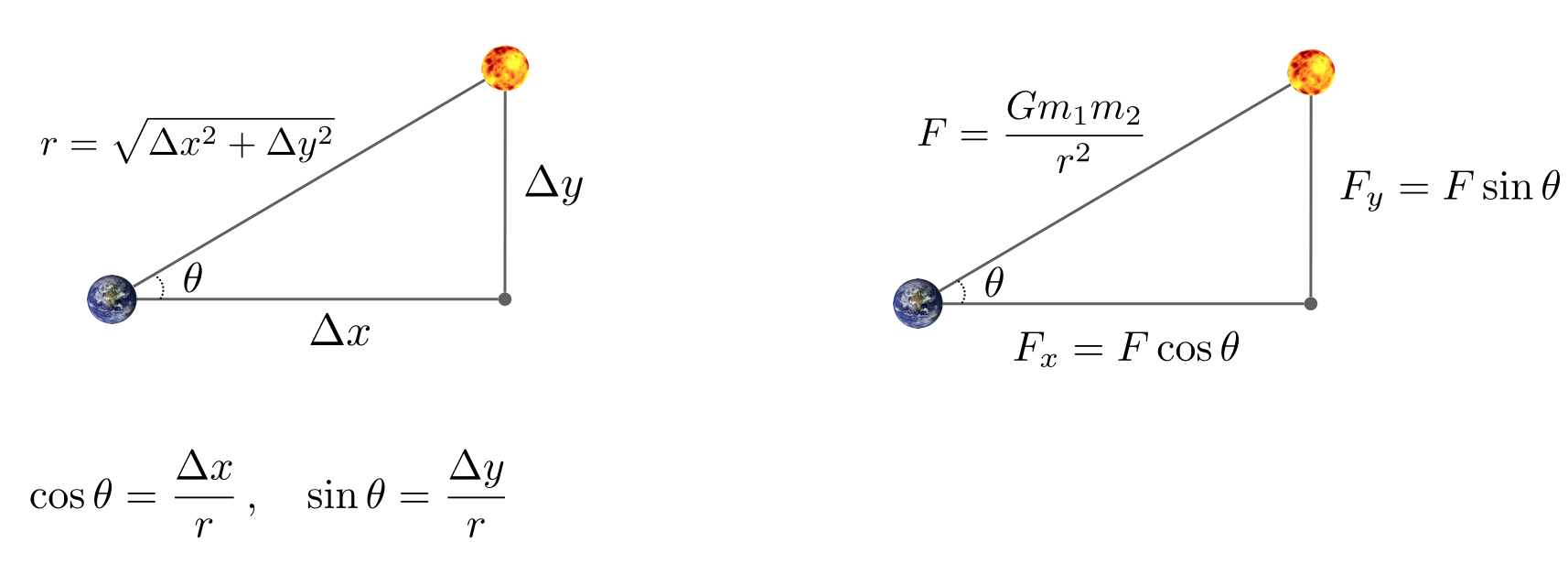COS 126 N-Body Simulation Programming Assignment Checklist

Write a program to simulate the motion of n particles in the plane, mutually affected by gravitational forces, and animate the results. Such methods are widely used in cosmology, semiconductors, and fluid dynamics to study complex physical systems. Scientists also apply the same techniques to other pairwise interactions including Coulombic, Biot–Savart, and van der Waals.

Context. In 1687, Isaac Newton formulated the principles governing the motion of two particles under the influence of their mutual gravitational attraction in his famous Principia. However, Newton was unable to solve the problem for three particles. Indeed, in general, solutions to systems of three or more particles must be approximated via numerical simulations.

Program specification. Write a program NBody.java that:

• Takes two double command-line arguments—the duration of the simulation (Τ) and the simulation time increment (Δt).

• Reads in the details of the universe to be simulated from standard input using StdIn, using several parallel arrays to store the data.

• Simulates the universe, starting at time t = 0.0, and continuing in Δt increments as long as t < Τ, using the leapfrog scheme described below.

• Animates the results to standard drawing using StdDraw.

• Prints the state of the universe at the end of the simulation (in the same format as the input file) to standard output using StdOut.

Input format. The input format is a text file that contains the information for a particular universe (in SI units).

• The first value is an integer n which represents the number of particles. The second value is a real number radius which represents the radius of the universe; it is used to determine the scaling of the drawing window (which displays particles with x- and y-coordinates between −radius and +radius).

• Next, there are n lines (one for each particle), with each line containing 6 values. The first two values are the x- and y-coordinates of the initial position; the next pair of values are the x- and y-components of the initial velocity; the fifth value is the mass; the last value is a String that is the name of an image file used to display the particle.

• The remainder of the file (optionally) contains a description of the universe, which your program must ignore.
As an example, planets.txt contains real data from part of our Solar System.Simulating the universe: the physics. We review the equations governing the motion of the particles, according to Newton's laws of motion and gravitation. Don't worry if your physics is a bit rusty; all of the necessary formulas are included below. We'll assume for now that the position (px, py) and velocity (vx, vy) of each particle is known. In order to model the dynamics of the system, we must know the net force exerted on each particle.

• Pairwise force. Newton's law of universal gravitation asserts that the strength of the gravitational force between two particles is given by the product of their masses divided by the square of the distance between them, scaled by the gravitational constant G (6.67 × 10−11 N·m2·kg−2). The pull of one particle towards another acts on the line between them. Since we are using Cartesian coordinates to represent the position of a particle, it is convenient to break up the force into its x- and y-components (Fx, Fy) as illustrated below.• Net force. The principle of superposition says that the net force acting on a particle in the x- or y-direction is the sum of the pairwise forces acting on the particle in that direction.

• Acceleration. Newton's second law of motion postulates that the accelerations in the x- and y-directions are given by: ax = Fx / m, ay = Fy / m.

Simulating the universe: the numerics.  We use the leapfrog finite difference approximation scheme to numerically integrate the above equations: this is the basis for most astrophysical simulations of gravitational systems. In the leapfrog scheme, we discretize time, and update the time variable t in increments of the time quantum Δt (measured in seconds). We maintain the position (px, py) and velocity (vx, vy) of each particle at each time step. The steps below illustrate how to evolve the positions and velocities of the particles.

• Step A (calculate the forces).  For each particle, calculate the net force (Fx, Fy) at the current time t acting on that particle using Newton's law of gravitation and the principle of superposition. Note that force is a vector (i.e., it has direction). In particular, Δx and Δy are signed (positive or negative). In the diagram above, when you compute the force the sun exerts on the earth, the sun is pulling the earth up (Δy positive) and to the right (Δx positive).

• Step B (update the velocities and positions).  For each particle:

1. Calculate its acceleration (ax, ay) at time t using the net force computed in Step A and Newton's second law of motion: ax = Fx / m, ay = Fy / m.

2. Calculate its new velocity (vx, vy) at the next time step by using the acceleration computed in (i) and the velocity from the old time step: Assuming the acceleration remains constant in this interval, the new velocity is (vx + Δt ax, vy + Δt ay).

3. Calculate its new position (px, py) at time t + Δt by using the velocity computed in (ii) and its old position at time t: Assuming the velocity remains constant in this interval, the new position is (px + Δt vx, py + Δt vy).

• Step C (draw the universe).  Draw each particle, using the position computed in Step B.
Do not interleave steps A and B; otherwise, you will be computing the forces at time t using the positions of some of the particles at time t and others at time t + Δt. The simulation is more accurate when Δt is very small, but this comes at the price of more computation.

Creating an animation. Draw each particle at its current position to standard drawing, and repeat this process at each time step until the designated stopping time. By displaying this sequence of snapshots (or frames) in rapid succession, you will create the illusion of movement. After each time step, draw the background image starfield.jpg; redraw all the particles in their new positions; and control the animation speed (about 40 frames per second looks good). You will call several methods from the StdDraw library.

Optional finishing touch. For a finishing touch, play the theme to 2001: A Space Odyssey using the StdAudio library and the audio file 2001.wav. It's a one-liner using the method StdAudio.play().

Compiling and executing your program. If you used our autoinstaller, you will use the commands `java-introcs` and `javac-introcs` (instead of `java` and `javac`) to compile and execute your programs. These versions provide access to the standard libraries.

To compile your program from the command line, type the following in your terminal application (Command Prompt or Terminal):

```% javac-introcs NBody.java
```

To execute your program from the command line, redirecting from the file planets.txt to standard input, type:

```% java-introcs NBody 157788000.0 25000.0 < planets.txt
```

Your browser can not display this movie.
Be sure that Javascript is enabled and that you have Flash 9.0.124 or better.

After the animation stops, your program must output the final state of the universe in the same format as the input, e.g.:
```5
2.50e11
1.4925e+11 -1.0467e+10  2.0872e+03  2.9723e+04  5.9740e+24    earth.gif
-1.1055e+11 -1.9868e+11  2.1060e+04 -1.1827e+04  6.4190e+23     mars.gif
-1.1708e+10 -5.7384e+10  4.6276e+04 -9.9541e+03  3.3020e+23  mercury.gif
2.1709e+05  3.0029e+07  4.5087e-02  5.1823e-02  1.9890e+30      sun.gif
6.9283e+10  8.2658e+10 -2.6894e+04  2.2585e+04  4.8690e+24    venus.gif
```

Getting started. Before you begin coding, do the following:

• Get familiar with the command line. If you used our autoinstaller [ Mac OS X · Windows ], your command line is ready to go. Review steps 4–6 on how to compile and execute a program from the command line. Use the javac-introcs and java-introcs commands to access the standard libraries.

• Get familiar with the standard libraries. To use our standard libraries, you need to have stdlib.jar available both to DrJava and your terminal application. If you used our autoinstaller, you should be all set. The standard libraries include StdIn (for reading data from standard input), StdOut (for writing data to standard output), StdDraw (for drawing results to standard drawing), and StdAudio (for sending sound to standard audio). The Java cheatsheet has a compact summary of these APIs.

• Download the data files. To test your program, you will need planets.txt and the accompanying image and sound files. The ZIP file nbody.zip contains these files, along with a readme.txt template and additional test universes.

Submission. Submit NBody.java and a completed readme.txt file.

Challenge for the bored. There are limitless opportunities for additional excitement and discovery here. Create an alternate universe (using the same input format). Try adding other features, such as supporting elastic or inelastic collisions. Or, make the simulation three-dimensional by doing calculations for x-, y-, and z-coordinates, then using the z-coordinate to vary the sizes of the planets. Add a rocket ship that launches from one planet and has to land on another. Allow the rocket ship to exert force with the consumption of fuel.